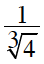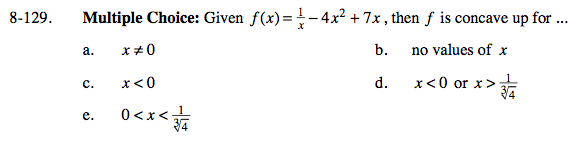### Home > CALC > Chapter 8 > Lesson 8.3.3 > Problem8-129

8-129.
1. Multiple Choice: Given f(x) =−4x2 + 7x, then f is concave up for … Homework Help ✎

1. x ≠ 0

2. no values of x

3. x < 0

4. x < 0 or x >5. 0 < x <Recall that candidates for points of inflection occur where the 2nd-derivative equals zero AND where the 2nd-derivative does not exist.

After you identify where f ''(x) = 0 and where f ''(x) = DNE, check the regions before, after and between those candidates: if f ''(x) > 0, then f(x) is concave up.